## THE CONCEPT OF THE LINKED LIST

### Introduction

When dealing with many problems we need a dynamic list, dynamic in the sense that the size requirement need not be known at compile time. Thus, the list may grow or shrink during runtime. A linked list is a data structure that is used to model such a dynamic list of data items, so the study of the linked lists as one of the data structures is important.

### Concept

An array is represented in memory using sequential mapping, which has the property that elements are fixed distance apart. But this has the following disadvantage: It makes insertion or deletion at any arbitrary position in an array a costly operation, because this involves the movement of some of the existing elements.

When we want to represent several lists by using arrays of varying size, either we have to represent each list using a separate array of maximum size or we have to represent each of the lists using one single array. The first one will lead to wastage of storage, and the second will involve a lot of data movement.

So we have to use an alternative representation to overcome these disadvantages. One alternative is a linked representation. In a linked representation, it is not necessary that the elements be at a fixed distance apart. Instead, we can place elements anywhere in memory, but to make it a part of the same list, an element is required to be linked with a previous element of the list. This can be done by storing the address of the next element in the previous element itself. This requires that every element be capable of holding the data as well as the address of the next element. Thus every element must be a structure with a minimum of two fields, one for holding the data value, which we call a data field, and the other for holding the address of the next element, which we call link field.

Therefore, a linked list is a list of elements in which the elements of the list can be placed anywhere in memory, and these elements are linked with each other using an explicit link field, that is, by storing the address of the next element in the link field of the previous element.

Program

Here is a program for building and printing the elements of the linked list:

``` # include
# include
struct node
{
int data;
};
struct node *insert(struct node *p, int n)
{
struct node *temp;
/* if the existing list is empty then insert a new node as the
starting node */
if(p==NULL)
{
p=(struct node *)malloc(sizeof(struct node)); /* creates new node
data value passes
as parameter */

if(p==NULL)
{
printf("Error
");
exit(0);
}
p-> data = n;
p-> link = p; /* makes the pointer pointing to itself because it
is a circular list*/
}
else
{
temp = p;
/* traverses the existing list to get the pointer to the last node of
it */
temp-> link = (struct node *)malloc(sizeof(struct node)); /*
creates new node using
data value passes as
parameter and puts its
of last node of the
existing list*/
{
printf("Error
");
exit(0);
}
temp-> data = n;
}
return (p);
}
void printlist ( struct node *p )
{
struct node *temp;
temp = p;
printf("The data values in the list are
");
if(p!= NULL)
{
do
{
printf("%d	",temp->data);
} while (temp!= p);
}
else
printf("The list is empty
");
}

void main()
{
int n;
int x;
struct node *start = NULL ;
printf("Enter the nodes to be created
");
scanf("%d",&n);
while ( n -- > 0 )
{
printf( "Enter the data values to be placed in a node
");
scanf("%d",&x);
start = insert ( start, x );
}
printf("The created list is
");
printlist ( start );
}
```

Explanation

1. This program uses a strategy of inserting a node in an existing list to get the list created. An insert function is used for this.
2. The insert function takes a pointer to an existing list as the first parameter, and a data value with which the new node is to be created as a second parameter, creates a new node by using the data value, appends it to the end of the list, and returns a pointer to the first node of the list.
3. Initially the list is empty, so the pointer to the starting node is NULL. Therefore, when insert is called first time, the new node created by the insert becomes the start node.
4. Subsequently, the insert traverses the list to get the pointer to the last node of the existing list, and puts the address of the newly created node in the link field of the last node, thereby appending the new node to the existing list.
5. The main function reads the value of the number of nodes in the list. Calls iterate that many times by going in a while loop to create the links with the specified number of nodes.

Points to Remember

1. Linked lists are used when the quantity of data is not known prior to execution.
2. In linked lists, data is stored in the form of nodes and at runtime, memory is allocated for creating nodes.
3. Due to overhead in memory allocation and deallocation, the speed of the program is lower.
4. The data is accessed using the starting pointer of the list.

## INSERTING A NODE BY USING RECURSIVE PROGRAMS

### Introduction

A linked list is a recursive data structure. A recursive data structure is a data structure that has the same form regardless of the size of the data. You can easily write recursive programs for such data structures.

Program

``` # include
# include
struct node
{
int data;
};
struct node *insert(struct node *p, int n)
{
struct node *temp;
if(p==NULL)
{
p=(struct node *)malloc(sizeof(struct node));
if(p==NULL)
{
printf("Error
");
exit(0);
}
p-> data = n;
}
else
recursive call */
return (p);
}
void printlist ( struct node *p )
{
printf("The data values in the list are
");
while (p!= NULL)
{
printf("%d	",p-> data);
}
}
void main()
{
int n;
int x;
struct node *start = NULL ;
printf("Enter the nodes to be created
");
scanf("%d",&n);
while ( n- > 0 )
{
printf( "Enter the data values to be placed in a node
");
scanf("%d",&x);
start = insert ( start, x );
}
printf("The created list is
");
printlist ( start );
}
```

Explanation

1. This recursive version also uses a strategy of inserting a node in an existing list to create the list.
2. An insert function is used to create the list. The insert function takes a pointer to an existing list as the first parameter, and a data value with which the new node is to be created as the second parameter. It creates the new node by using the data value, then appends it to the end of the list. It then returns a pointer to the first node of the list.
3. Initially, the list is empty, so the pointer to the starting node is NULL. Therefore, when insert is called the first time, the new node created by the insert function becomes the start node.
4. Subsequently, the insert function traverses the list by recursively calling itself.
5. The recursion terminates when it creates a new node with the supplied data value and appends it to the end of the list.

Points to Remember

1. A linked list has a recursive data structure.
2. Writing recursive programs for such structures is programmatically convenient.

## SORTING AND REVERSING A LINKED LIST

### Introduction

To sort a linked list, first we traverse the list searching for the node with a minimum data value. Then we remove that node and append it to another list which is initially empty. We repeat this process with the remaining list until the list becomes empty, and at the end, we return a pointer to the beginning of the list to which all the nodes are moved, as shown in Figure 20.1.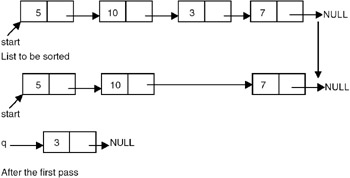Figure 20.1: Sorting of a linked list.

To reverse a list, we maintain a pointer each to the previous and the next node, then we make the link field of the current node point to the previous, make the previous equal to the current, and the current equal to the next, as shown in Figure 20.2.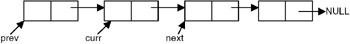Figure 20.2: A linked list showing the previous, current, and next nodes at some point during reversal process.

Therefore, the code needed to reverse the list is

```Prev = NULL;
While (curr != NULL)
{
Prev = curr;
Curr = next;
}
```

Program

```# include
# include
struct node
{
int data;
};
struct node *insert(struct node *p, int n)
{
struct node *temp;
if(p==NULL)
{
p=(struct node *)malloc(sizeof(struct node));
if(p==NULL)
{
printf("Error
");
exit(0);
}
p-> data = n;
}
else
{
temp = p;
temp-> link = (struct node *)malloc(sizeof(struct node));
{
printf("Error
");
exit(0);
}
temp-> data = n;
}
return(p);
}

void printlist ( struct node *p )
{
printf("The data values in the list are
");
while (p!= NULL)
{
printf("%d	",p-> data);
}
}

/* a function to sort reverse list */
struct node *reverse(struct node *p)
{
struct node *prev, *curr;
prev = NULL;
curr = p;
while (curr != NULL)
{
prev = curr;
curr = p;
}
return(prev);
}
/* a function to sort a list */
struct node *sortlist(struct node *p)
{
struct node *temp1,*temp2,*min,*prev,*q;
q = NULL;
while(p != NULL)
{
prev = NULL;
min = temp1 = p;
while ( temp2 != NULL )
{
if(min -> data > temp2 -> data)
{
min = temp2;
prev = temp1;
}
temp1 = temp2;
}
if(prev == NULL)
else
if( q == NULL)
q = min; /* moves the node with lowest data value in the list
pointed to by p to the list
pointed to by q as a first node*/
else
{
temp1 = q;
/* traverses the list pointed to by q to get pointer to its
last node */
while( temp1 -> link != NULL)
temp1 -> link = min; /* moves the node with lowest data value
in the list pointed to
by p to the list pointed to by q at the end of list pointed by
q*/
}
}
return (q);
}

void main()
{
int n;
int x;
struct node *start = NULL ;
printf("Enter the nodes to be created
");
scanf("%d",&n);
while ( n- > 0 )
{
printf( "Enter the data values to be placed in a
node
");
scanf("%d",&x);
start = insert ( start,x);
}
printf("The created list is
");
printlist ( start );
start = sortlist(start);
printf("The sorted list is
");
printlist ( start );
start = reverse(start);
printf("The reversed list is
");
printlist ( start );
}
```

Explanation

The working of the sorting function on an example list is shown in Figure 20.3.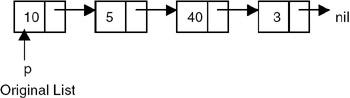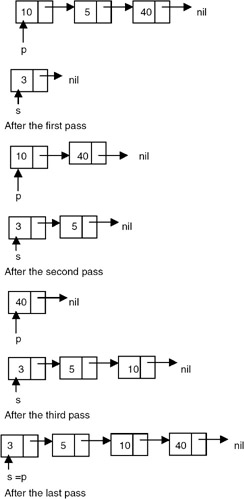Figure 20.3: Sorting of a linked list.

The working of a reverse function is shown in Figure 20.4.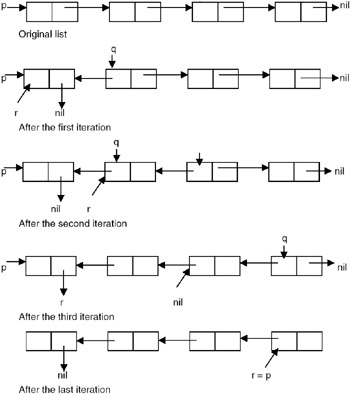Figure 20.4: Reversal of a list.

## DELETING THE SPECIFIED NODE IN A SINGLY LINKED LIST

### Introduction

To delete a node, first we determine the node number to be deleted (this is based on the assumption that the nodes of the list are numbered serially from 1 to n). The list is then traversed to get a pointer to the node whose number is given, as well as a pointer to a node that appears before the node to be deleted. Then the link field of the node that appears before the node to be deleted is made to point to the node that appears after the node to be deleted, and the node to be deleted is freed. Figures 20.5 and 20.6 show the list before and after deletion, respectively.

Program

```# include
# include
struct node *delet ( struct node *, int );
int length ( struct node * );
struct node
{
int data;
};
struct node *insert(struct node *p, int n)
{
struct node *temp;
if(p==NULL)
{
p=(struct node *)malloc(sizeof(struct node));
if(p==NULL)
{
printf("Error
");
exit(0);
}
p-> data = n;
}
else
{
temp = p;
temp-> link = (struct node *)malloc(sizeof(struct node));
{
printf("Error
");
exit(0);
}
temp-> data = n;
}
return (p);
}

void printlist ( struct node *p )
{
printf("The data values in the list are
");
while (p!= NULL)
{
printf("%d	",p-> data);
}
}

void main()
{
int n;
int x;
struct node *start = NULL;
printf("Enter the nodes to be created
");
scanf("%d",&n);
while ( n- > 0 )
{
printf( "Enter the data values to be placed in a node
");
scanf("%d",&x);
start = insert ( start, x );
}
printf(" The list before deletion id
");
printlist ( start );
printf("%
Enter the node no
");
scanf ( " %d",&n);
start = delet (start , n );
printf(" The list after deletion is
");
printlist ( start );
}

/* a function to delete the specified node*/
struct node *delet ( struct node *p, int node_no )
{

struct node *prev, *curr ;
int i;

if (p == NULL )
{
printf("There is no node to be deleted
");
}
else
{
if ( node_no > length (p))
{
printf("Error
");
}
else
{
prev = NULL;
curr = p;
i = 1 ;
while ( i < node_no )
{
prev = curr;
i = i+1;
}
if ( prev == NULL )
{
free ( curr );
}
else
{
free ( curr );
}
}
}
return(p);
}
/* a function to compute the length of a linked list */
int length ( struct node *p )
{
int count = 0 ;
while ( p != NULL )
{
count++;
}
return ( count ) ;
}
```

Explanation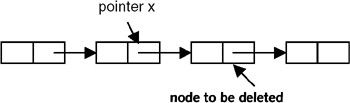Figure 20.5: Before deletion.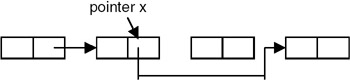Figure 20.6: After deletion.

## INSERTING A NODE AFTER THE SPECIFIED NODE IN A SINGLY LINKED LIST

### Introduction

To insert a new node after the specified node, first we get the number of the node in an existing list after which the new node is to be inserted. This is based on the assumption that the nodes of the list are numbered serially from 1 to n. The list is then traversed to get a pointer to the node, whose number is given. If this pointer is x, then the link field of the new node is made to point to the node pointed to by x, and the link field of the node pointed to by x is made to point to the new node. Figures 20.7 and 20.8 show the list before and after the insertion of the node, respectively.

Program

``` # include
# include
int length ( struct node * );
struct node
{
int data;
};

/* a function which appends a new node to an existing list used for
building a list */
struct node *insert(struct node *p, int n)
{
struct node *temp;
if(p==NULL)
{
p=(struct node *)malloc(sizeof(struct node));
if(p==NULL)
{
printf("Error
");
exit(0);
}
p-> data = n;
}
else
{
temp = p;
temp-> link = (struct node *)malloc(sizeof(struct node));
{
printf("Error
");
exit(0);
}
temp-> data = n;
}
return (p);
}
/* a function which inserts a newly created node after the specified
node */
struct node * newinsert ( struct node *p, int node_no, int value )
{
struct node *temp, * temp1;
int i;
if ( node_no <= 0 || node_no > length (p))
{
printf("Error! the specified node does not exist
");
exit(0);
}
if ( node_no == 0)
{
temp = ( struct node * )malloc ( sizeof ( struct node ));
if ( temp == NULL )
{
printf( " Cannot allocate
");
exit (0);
}
temp -> data = value;
p = temp ;
}
else
{
temp = p ;
i = 1;
while ( i < node_no )
{
i = i+1;
}
temp1 = ( struct node * )malloc ( sizeof(struct node));
if ( temp == NULL )
{
printf ("Cannot allocate
");
exit(0)
}
temp1 -> data = value ;
}
return (p);
}

void printlist ( struct node *p )
{
printf("The data values in the list are
");
while (p!= NULL)
{
printf("%d	",p-> data);
}
}
void main ()
{
int n;
int x;
struct node *start = NULL;
printf("Enter the nodes to be created
");
scanf("%d",&n);
while ( n- > 0 )
{
printf( "Enter the data values to be placed in a node
");
scanf("%d",&x);
start = insert ( start, x );
}
printf(" The list before deletion is
");
printlist ( start );
printf("
Enter the node no after which the insertion is to be
done
");
scanf ( " %d",&n);
printf("Enter the value of the node
");
scanf("%d",&x);
start = newinsert(start,n,x);
printf("The list after insertion is
");
printlist(start);
}
```

Explanation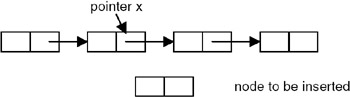Figure 20.7: Before insertion.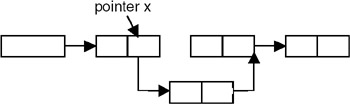Figure 20.8: After insertion.

## INSERTING A NEW NODE IN A SORTED LIST

### Introduction

To insert a new node into an already sorted list, we compare the data value of the node to be inserted with the data values of the nodes in the list starting from the first node. This is continued until we get a pointer to the node that appears immediately before the node in the list whose data value is greater than the data value of the node to be inserted.

Program

Here is a complete program to insert an element in a sorted list of elements using the linked list representation so that after insertion, it will remain a sorted list.

```# include
# include
struct node
{
int data;
};
struct node *insert(struct node *, int);
struct node *sinsert(struct node*, int );
void printlist ( struct node * );
struct node *sortlist(struct node *);

struct node *insert(struct node *p, int n)
{
struct node *temp;
if(p==NULL)
{
p=(struct node *)malloc(sizeof(struct node));
if(p==NULL)
{
printf("Error
");
exit(0);
}
p-> data = n;
}
else
{
temp = p;
temp-> link = (struct node *)malloc(sizeof(struct node));
{
printf("Error
");
exit(0);
}
temp-> data = n;
}
return (p);
}

void printlist ( struct node *p )
{
printf("The data values in the list are
");
while (p!= NULL)
{
printf("%d	",p-> data);
}
}

/* a function to sort a list */
struct node *sortlist(struct node *p)
{
struct node *temp1,*temp2,*min,*prev,*q;
q = NULL;
while(p != NULL)
{
prev = NULL;
min = temp1 = p;
while ( temp2 != NULL )
{
if(min -> data > temp2 -> data)
{
min = temp2;
prev = temp1;
}
temp1 = temp2;
}
if(prev == NULL)
else
if( q == NULL)
q = min; /* moves the node with lowest data value in the list
pointed to by p to the list
pointed to by q as a first node*/
else
{
temp1 = q;
/* traverses the list pointed to by q to get pointer to its
last node */
while( temp1 -> link != NULL)
temp1 -> link = min; /* moves the node with lowest data value
in the list pointed to
by p to the list pointed to by q at the end of list pointed by
q*/
}
}
return (q);
}

/* a function to insert a node with data value n in a sorted list
pointed to by p*/
struct node *sinsert(struct node *p, int n)
{
struct node *curr, *prev;
curr =p;
prev = NULL;
while(curr ->data < n)
{
prev = curr;
}
if ( prev == NULL) /* the element is to be inserted at the start of
the list because
it is less than the data value of the first node*/
{
curr = (struct node *) malloc(sizeof(struct node));
if( curr == NULL)
{
printf("error cannot allocate
");
exit(0);
}
curr->data = n;
p = curr;
}
else
{
curr->data = n;
}
return(p);
}

void main()
{
int n;
int x;
struct node *start = NULL ;
printf("Enter the nodes to be created
");
scanf("%d",&n);
while ( n-- > 0 )
{
printf( "Enter the data values to be placed in a node
");
scanf("%d",&x);
start = insert ( start,x);
}
printf("The created list is
");
printlist ( start );
start = sortlist(start);
printf("The sorted list is
");
printlist ( start );
printf("Enter the value to be inserted
");
scanf("%d",&n);
start = sinsert(start,n);
printf("The list after insertion is
");
printlist ( start );
}
```

Explanation

1. If this pointer is prev, then prev is checked for a NULL value.
2. If prev is NULL, then the new node is created and inserted as the first node in the list.
3. When prev is not NULL, then a new node is created and inserted after the node pointed by prev, as shown in Figure 20.9.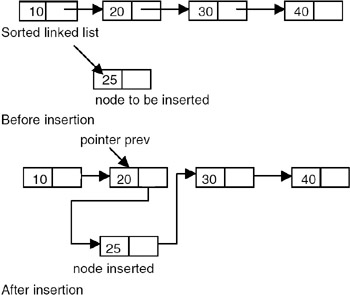Figure 20.9: Insertion in a sorted list.

## COUNTING THE NUMBER OF NODES OF A LINKED LIST

### Introduction

Counting the number of nodes of a singly linked list requires maintaining a counter that is initialized to 0 and incremented by 1 each time a node is encountered in the process of traversing a list from the start.

Here is a complete program that counts the number of nodes in a singly linked chain p, where p is a pointer to the first node in the list.

Program

```# include
# include
struct node
{
int data;
};
struct node *insert(struct node *, int);
int nodecount(struct node*);
void printlist ( struct node * );

struct node *insert(struct node *p, int n)
{
struct node *temp;
if(p==NULL)
{
p=(struct node *)malloc(sizeof(struct node));
if(p==NULL)
{
printf("Error
");
exit(0);
}
p-> data = n;
}
else
{
temp = p;
temp-> link = (struct node *)malloc(sizeof(struct node));
{
printf("Error
");
exit(0);
}
temp-> data = n;
}
return (p);
}

void printlist ( struct node *p )
{
printf("The data values in the list are
");
while (p!= NULL)
{
printf("%d	",p-> data);
}
}

/* A function to count the number of nodes in a singly linked list */
int nodecount (struct node *p )
{
int count=0;
while (p != NULL)
{
count ++;
}
return(count);
}

void main()
{
int n;
int x;
struct node *start = NULL ;
printf("Enter the nodes to be created
");
scanf("%d",&n);
while ( n-- > 0 )
{
printf( "Enter the data values to be placed in a node
");
scanf("%d",&x);
start = insert ( start,x);
}
printf("The created list is
");
printlist ( start );
n = nodecount(start);
printf("The number of nodes in a list are: %d
",n);

}
```

## MERGING OF TWO SORTED LISTS

### Introduction

Merging of two sorted lists involves traversing the given lists and comparing the data values stored in the nodes in the process of traversing.

If p and q are the pointers to the sorted lists to be merged, then we compare the data value stored in the first node of the list pointed to by p with the data value stored in the first node of the list pointed to by q. And, if the data value in the first node of the list pointed to by p is less than the data value in the first node of the list pointed to by q, make the first node of the resultant/merged list to be the first node of the list pointed to by p, and advance the pointer p to make it point to the next node in the same list.

If the data value in the first node of the list pointed to by p is greater than the data value in the first node of the list pointed to by q, make the first node of the resultant/merged list to be the first node of the list pointed to by q, and advance the pointer q to make it point to the next node in the same list.

Repeat this procedure until either p or q becomes NULL. When one of the two lists becomes empty, append the remaining nodes in the non-empty list to the resultant list.

Program

```
# include
# include
struct node
{
int data;
};

struct node *merge (struct node *, struct node *);
struct node *insert(struct node *p, int n)
{
struct node *temp;
if(p==NULL)
{
p=(struct node *)malloc(sizeof(struct node));
if(p==NULL)
{
printf("Error
");
exit(0);
}
p-> data = n;
}
else
{
temp = p;
temp-> link = (struct node *)malloc(sizeof(struct node));
{
printf("Error
");
exit(0);
}
temp-> data = n;
}
return (p);
}

void printlist ( struct node *p )
{
printf("The data values in the list are
");
while (p!= NULL)
{
printf("%d	",p-> data);
}
}
/* a function to sort a list */
struct node *sortlist(struct node *p)
{
struct node *temp1,*temp2,*min,*prev,*q;
q = NULL;
while(p != NULL)
{
prev = NULL;
min = temp1 = p;
while ( temp2 != NULL )
{
if(min -> data > temp2 -> data)
{
min = temp2;
prev = temp1;
}
temp1 = temp2;
}
if(prev == NULL)
else
if( q == NULL)
q = min; /* moves the node with lowest data value in the list
pointed to by p to the list
pointed to by q as a first node*/
else
{
temp1 = q;
/* traverses the list pointed to by q to get pointer to its
last node */
while( temp1 -> link != NULL)
temp1 -> link = min; /* moves the node with lowest data value
in the list pointed to
by p to the list pointed to by q at the end of list pointed by
q*/
}
}
return (q);
}

void main()
{
int n;
int x;
struct node *start1 = NULL ;
struct node *start2 = NULL;
struct node *start3 = NULL;
/* The following code creates and sorts the first list */
printf("Enter the number of nodes in the first list
");
scanf("%d",&n);
while ( n-- > 0 )
{
printf( "Enter the data value to be placed in a node
");
scanf("%d",&x);
start1 = insert ( start1,x);
}
printf("The first list is
");
printlist ( start1);
start1 = sortlist(start1);
printf("The sorted list1 is
");
printlist ( start1 );
/* the following creates and sorts the second list*/
printf("Enter the number of nodes in the second list
");
scanf("%d",&n);
while ( n-- > 0 )
{
printf( "Enter the data value to be placed in a node
");
scanf("%d",&x);
start2 = insert ( start2,x);
}
printf("The second list is
");
printlist ( start2);
start2 = sortlist(start2);
printf("The sorted list2 is
");
printlist ( start2 );
start3 = merge(start1,start2);
printf("The merged list is
");
printlist ( start3);
}

/* A function to merge two sorted lists */
struct node *merge (struct node *p, struct node *q)
{
struct node *r=NULL,*temp;
if (p == NULL)
r = q;
else
if(q == NULL)
r = p;
else
{
if (p->data < q->data )
{
r = p;
temp = p;
}
else
{
r = q;
temp =q;
}
while((p!= NULL) && (q != NULL))
{
if (p->data < q->data)
{
}
else
{
}
}
if (p!= NULL)
if (q != NULL)
}
return( r) ;
}
```

Explanation

If the following lists are given as input, then what would be the output of the program after each pass? This is shown here: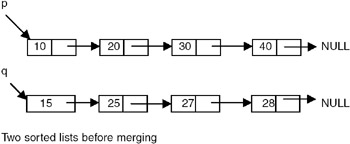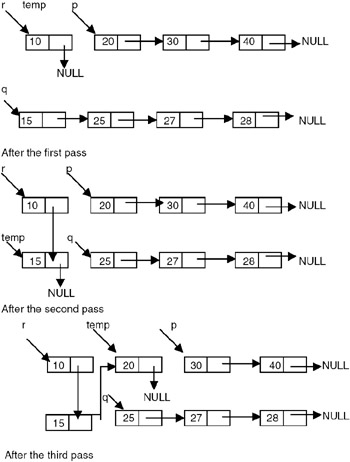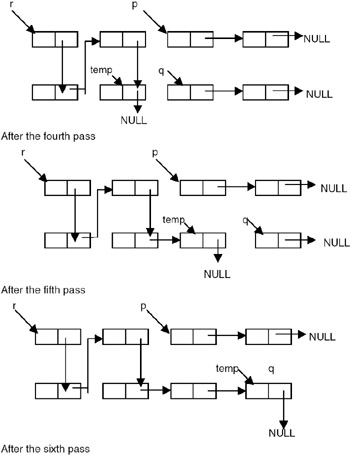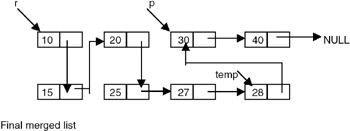### Introduction

Erasing a linked list involves traversing the list starting from the first node, freeing the storage allocated to the nodes, and then setting the pointer to the list to NULL. If p is a pointer to the start of the list, the actions specified through the following code will erase the list:

```while(p != NULL)
{
temp = p;
free(t);
}
```

But a better strategy of erasing a list is to mark all the nodes of the list to be erased as free nodes without actually freeing the storage of these nodes. That means to maintain this list, a list of free nodes, so that if a new node is required it can be obtained from this list of free nodes.

Program

Here is a complete program that erases a list pointed to by p by adding the nodes of a list pointed by p to the free list.

```# include
# include
struct node
{
int data;
};
struct node *insert(struct node *, int);
void erase(struct node **,struct node **);
void printlist ( struct node * );

void erase (struct node **p, struct node **free)
{
struct node *temp;
temp = *p;
*free = *p;
*p = NULL;
}

struct node *insert(struct node *p, int n)
{
struct node *temp;
if(p==NULL)
{
p=(struct node *)malloc(sizeof(struct node));
if(p==NULL)
{
printf("Error
");
exit(0);
}
p-> data = n;
}
else
{
temp = p;
temp-> link = (struct node *)malloc(sizeof(struct node));
{
printf("Error
");
exit(0);
}
temp-> data = n;
}
return (p);
}

void printlist ( struct node *p )
{
printf("The data values in the list are
");
while (p!= NULL)
{
printf("%d	",p-> data);
}
}

void main()
{
int n;
int x;
struct node *start = NULL ;
struct node *free=NULL;

/* this code will create a free list for the test purpose*/
printf("Enter the number of nodes in the initial free list
");
scanf("%d",&n);
while ( n-- > 0 )
{
printf( "Enter the data values to be placed in a node
");
scanf("%d",&x);
free = insert ( free,x);
}

/* this code will create a list to be erased*/
printf("Enter the number of nodes in the list to be created for
erasing
");
scanf("%d",&n);
while ( n-- > 0 )
{
printf( "Enter the data values to be placed in a node
");
scanf("%d",&x);
start = insert ( start,x);
}
printf("The free list islist is:
");
printlist ( free );
printf("The list to be erased is:
");
printlist ( start);
erase(&start,&free);
printf("The free list after adding all the nodes from the list to
be erased is:
");
printlist ( free );
}
```

Explanation

The method of erasing a list requires adding all the nodes of the list to be erased to the list of free nodes, as shown here.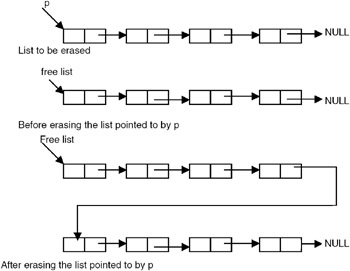## POLYNOMIAL REPRESENTATION

### Introduction

One of the problems that a linked list can deal with is manipulation of symbolic polynomials. By symbolic, we mean that a polynomial is viewed as a list of coefficients and exponents. For example, the polynomial

3x2+2x+4,

can be viewed as list of the following pairs

(3,2),(2,1),(4,0)

Therefore, we can use a linked list in which each node will have three fields, as shown in Figure 20.10.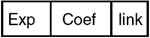A polynomial 10x4 + 5x2 + 1 can be represented as shown here:Figure 20.10: Polynomial representation.

The procedure to add these two polynomials using the linked list is in the following program.

Program

```# include
# include
struct pnode
{
int exp;
double coeff;
};

struct pnode *insert(struct pnode *, int,double);
void printlist ( struct pnode * );
struct pnode *polyadd(struct pnode *, struct pnode *);
struct pnode *sortlist(struct pnode *);

struct pnode *insert(struct pnode *p, int e,double c)
{
struct pnode *temp;
if(p==NULL)
{
p=(struct pnode *)malloc(sizeof(struct pnode));
if(p==NULL)
{
printf("Error
");
exit(0);
}
p-> exp = e;
p->coeff = c;
}
else
{
temp = p;
temp-> link = (struct pnode *)malloc(sizeof(struct pnode));
{
printf("Error
");
exit(0);
}
temp-> exp = e;
temp->coeff = c;
}
return (p);
}

/* a function to sort a list */
struct pnode *sortlist(struct pnode *p)
{
struct pnode *temp1,*temp2,*max,*prev,*q;
q = NULL;
while(p != NULL)
{
prev = NULL;
max = temp1 = p;
while ( temp2 != NULL )
{
if(max -> exp < temp2 -> exp)
{
max = temp2;
prev = temp1;
}
temp1 = temp2;
}
if(prev == NULL)
else
if( q == NULL)
q = max; /* moves the node with highest data value in the list
pointed to by p to the list
pointed to by q as a first node*/
else
{
temp1 = q;
/* traverses the list pointed to by q to get pointer to its
last node */
while( temp1 -> link != NULL)
temp1 -> link = max; /* moves the node with highest data value
in the list pointed to
by p to the list pointed to by q at the end of list pointed by
q*/

}
}
return (q);
}
/* A function to add two polynomials */
struct pnode *polyadd(struct pnode *p, struct pnode *q)
{
struct pnode *r = NULL;
int e;
double c;
while((p!=NULL) && (q != NULL))
{
if(p->exp > q->exp)
{
r = insert(r,p->exp,p->coeff);
}
else
if(p->exp < q->exp)
{
r = insert(r,q->exp,q->coeff);
}
else
{
c = p->coeff + q->coeff;
e = q->exp;
r = insert( r, e,c);
}
while(p != NULL)
{
r = insert( r, p->exp,p->coeff);
}
while(q!=NULL)
{
r = insert( r, q->exp,q->coeff);
}
return(r);
}

void printlist ( struct pnode *p )
{
printf("The polynomial is
");
while (p!= NULL)
{
printf("%d %lf	",p-> exp,p->coeff);
}
}
void main()
{
int e;
int n,i;
double c;
struct pnode *poly1 = NULL ;
struct pnode *poly2=NULL;
struct pnode *result;
printf("Enter the terms in the polynomial1
");
scanf("%d",&n);
i=1;
while ( n-- > 0 )
{
printf( "Enter the exponent and coefficient of the term number
%d
",i);
scanf("%d %lf",&e,&c);
poly1 = insert ( poly1,e,c);
}
printf("Enter the terms in the polynomial2
");
scanf("%d",&n);
i=1;
while ( n-- > 0 )
{
printf( "Enter the exponent and coefficient of the term number
%d
",i);
scanf("%d %lf",&e,&c);
poly2 = insert ( poly2,e,c);
}
poly1 = sortlist(poly1);
poly2 = sortlist(poly2);
printf("The polynomial 1 is
");
printlist ( poly1 );
printf("The polynomial 2 is
");
printlist ( poly2 );
");
printlist ( result );
}
```

Explanation

1. If the polynomials to be added have n and m terms, respectively, then the linked list representation of these polynomials contains m and n terms, respectively.
2. Since polyadd traverses each of these lists, sequentially, the maximum number of iterations that polyadd will make will not be more than m + n. So the computation time of polyadd is O(m + n).

## REPRESENTATION OF SPARSE MATRICES

### Introduction

A matrix is a two-dimensional data object made of m rows and n columns, therefore having m ´ n values. When m=n, we call it a square matrix.

The most natural representation is to use two-dimensional array A[m][n] and access the element of ith row and jth column as A[i][j]. If a large number of elements of the matrix are zero elements, then it is called a sparse matrix.

Representing a sparse matrix by using a two-dimensional array leads to the wastage of a substantial amount of space. Therefore, an alternative representation must be used for sparse matrices. One such representation is to store only non- zero elements along with their row positions and column positions. That means representing every non-zero element by using triples (i, j, value), where i is a row position and j is a column position, and store these triples in a linear list. It is possible to arrange these triples in the increasing order of row indices, and for the same row index in the increasing order of column indices. Each triple (i,j,value) can be represented by using a node having four fields as shown in the following:

```Struct snode{
Int row,col,val;
Struct snode *next;
};
```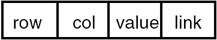So a sparse matrix can be represented using a list of such nodes, one per non–zero element of the matrix. For example, consider the sparse matrix shown in Figure 20.11.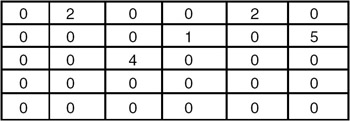Figure 20.11: A sparse matrix.

This matrix can be represented using the linked list shown in Figure 20.12.Figure 20.12: Linked list representation of sparse matrix of Figure 20.11.

Program

Here is a program for the addition of two sparse matrices:

```# include
# include
struct snode
{
int row,col,val;
};

struct snode *insert(struct snode *, int,int,int);
void printlist ( struct snode * );
struct snode *sadd(struct snode *, struct snode *);
//struct pnode *sortlist(struct pnode *);

struct snode *insert(struct snode *p, int r,int c,int val)
{
struct snode *temp;
if(p==NULL)
{
p=(struct snode *)malloc(sizeof(struct snode));
if(p==NULL)
{
printf("Error
");
exit(0);
}
p->row = r;
p->col = c;
p->val = val;
}
else
{
temp = p;
temp-> link = (struct snode *)malloc(sizeof(struct snode));
{
printf("Error
");
exit(0);
}
temp-> row = r;
temp->col= c;
temp->val=val;
}
return (p);
}

/* A function to add two sparse matrices */
struct snode *sadd(struct snode *p, struct snode *q)
{
struct snode *r = NULL;
int val;
while((p!=NULL) && (q != NULL))
{
if(p->row < q->row)
{
r = insert(r,p->row,p->col,p->val);
}
else
if(p->row > q->row)
{
r = insert(r,q->row,q->col,q->val);
}
else
if( p->col < q->col)
{
r = insert(r,p->row,p->col,p->val);
}
else
if(p->col > q->col)
{
r = insert(r,q->row,q->col,q->val);
}
else
{
val = p->val + q->val;
r = insert( r, p->row,p->col,val);
}
}
while(p != NULL)
{
r = insert( r, p->row ,p->col,p->val);
}
while(q!=NULL)
{
r = insert( r, q->row ,q->col,q->val);
}
return(r);
}

void printlist ( struct snode *p )
{
printf("The resultant sparse matrix is
");
while (p!= NULL)
{
printf("%d %d % d
",p-> row,p->col,p->val);
}
}
void main()
{
int r,n,c,val;
struct snode *s1 = NULL ;
struct snode *s2=NULL;
struct snode *result = NULL;
printf("Enter the number of non-zero terms in the sparse matrix1
");
scanf("%d",&n);
printf("Enter the terms in the sparse matrix1 in the increasing
order of row indices and for the same row index in the increasing order of
row indices and for the same row index in the increasing order of column
indices
");
while ( n-- > 0 )
{
printf( "Enter the row number, column number, and value
");
scanf("%d %d%d",&r,&c,&val);
s1 = insert ( s1,r,c,val);
}
printf("Enter the number of non-zero terms in the sparse matrix1
");
scanf("%d",&n);
printf("Enter the terms in the sparse matrix2 in the increasing
order of row indices and for the same row index in the increasing order of
row indices and for the same row index in the increasing order of column
indices
");
while ( n-- > 0 )
{
printf( "Enter the row number, column number, and value
");
scanf("%d %d%d",&r,&c,&val);
s2 = insert ( s2,r,c,val);
}
");
printlist ( result );
}
```

Explanation

1. In order to add two sparse matrices represented using the sorted linked lists as shown in the preceding program, the lists are traversed until the end of one of the lists is reached.
2. In the process of traversal, the row indices stored in the nodes of these lists are compared. If they don't match, a new node is created and inserted into the resultant list by copying the contents of a node with a lower value of row index. The pointer in the list containing a node with a lower value of row index is advanced to make it point to the next node.
3. If the row indices match, column indices for the corresponding row positions are compared. If they don't match, a new node is created and inserted into the resultant list by copying the contents of a node with a lower value of column index. The pointer in the list containing a node with a lower value of column index is advanced to make it point to the next node.
4. If the column indices match, a new node is created and inserted into the resultant list by copying the row and column indices from any of the nodes and the value equal to the sum of the values in the two nodes.
5. After this, the pointers in both the lists are advanced to make them point to the next nodes in the respective lists. This process is repeated in each iteration. After reaching the end of any one of the lists, the iterations come to an end and the remaining nodes in the list whose end has not been reached are copied, as it is in the resultant list.

### Example

Consider the following sparse matrices: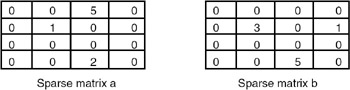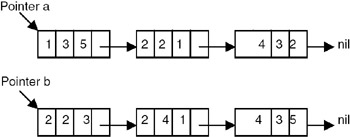If the procedure sadd is applied to the above linked list representations then we get the resultant list, as shown in Figure 20.13.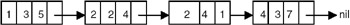Figure 20.13: Result of application of the procedure sadd.

This resultant list represents the matrix shown below: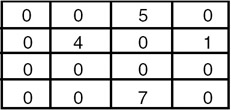This matrix is an addition of the matrices of a and b, respectively.

### Points to Remember

1. If the sparse matrices to be added have n and m non-zero terms, respectively, then the linked list representation of these sparse matrices contains m and n terms, respectively.
2. Since sadd traverses each of these lists sequentially, the maximum number of iterations that sadd will make will not be more than m+n. So the computation time of sadd is O(m+n).

### Introduction

A circular list is a list in which the link field of the last node is made to point to the start/first node of the list, as shown in Figure 20.14.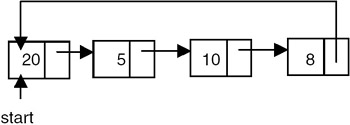Figure 20.14: A circular list.

In the case of circular lists, the empty list also should be circular. So to represent a circular list that is empty, it is required to use a header node or a head-node whose data field contents are irrelevant, as shown in Figure 20.15.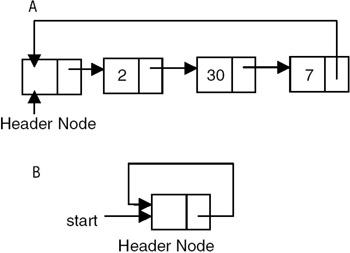Figure 20.15: (A) A circular list with head node, (B) an empty circular list.

Program

Here is a program for building and printing the elements of the circular linked list.

```# include
# include
struct node
{
int data;
};
struct node *insert(struct node *p, int n)
{
struct node *temp;
/* if the existing list is empty then insert a new node as the
starting node */
if(p==NULL)
{
p=(struct node *)malloc(sizeof(struct node)); /* creates new
node data value passes
as parameter */
if(p==NULL)
{
printf("Error
");
exit(0);
}
p-> data = n;
p-> link = p; /* makes the pointer pointing to itself because it
is a circular list*/
}
else
{
temp = p;
/* traverses the existing list to get the pointer to the last node of
it */
temp-> link = (struct node *)malloc(sizeof(struct node)); /*
creates new node using
data value passes as
parameter and puts its
of last node of the
existing list*/
{
printf("Error
");
}
exit(0);
temp-> data = n;
}
return (p);
}
void printlist ( struct node *p )
{
struct node *temp;
temp = p;
printf("The data values in the list are
");
if(p!= NULL)
{
do
{
printf(%d	",temp->data);
} while (temp!= p)
}
else
printf("The list is empty
");
}

void main()
{
int n;
int x;
struct node *start = NULL ;
printf("Enter the nodes to be created
");
scanf("%d",&n);
while ( n- > 0 )
{
printf( "Enter the data values to be placed in a
node
");
scanf("%d",&x);
start = insert ( start, x );
}
printf("The created list is
");
printlist ( start );
}
```

Explanation

The program appends a new node to the existing list (that is, it inserts a new node in the existing list at the end), and it makes the link field of the newly inserted node point to the start or first node of the list. This ensures that the link field of the last node always points to the starting node of the list.

## SPLITTING A LIST WITH 2N NODES INTO TWO SEPARATE AND EQUAL LISTS

### Introduction

If the circular linked list has 10 nodes, then the two lists have 5 nodes each. The procedure for splitting a circular list with 2n nodes into two equal circular lists is given here:

Program

```# include
# include
struct node
{
int data;
};
void split(struct node *p, struct node **q, int n)
{
struct node *temp;
int i =1;
temp = p;
while( i < n)

{
i++;
}
temp = *q;
}

struct node *insert(struct node *p, int n)
{
struct node *temp;
/* if the existing list is empty then insert a new node as the
starting node */
if(p==NULL)
{
p=(struct node *)malloc(sizeof(struct node)); /* creates new node
data value passes
as parameter */

if(p==NULL)
{
printf("Error
");
exit(0);
}
p-> data = n;
p-> link = p; /* makes the pointer point to itself because it is
a circular list*/
}
else
{
temp = p;
/* traverses the existing list to get the pointer to the last node of
it */
temp-> link = (struct node *)malloc(sizeof(struct node)); /*
creates new node using
data value passes as
parameter and puts its
of last node of the
existing list*/
{
printf("Error
");
exit(0);
}
temp-> data = n;
}
return (p);
}

void printlist ( struct node *p )
{
struct node *temp;
temp = p;
printf("The data values in the list are
");
if(p!= NULL)
do
{
printf("%d	",temp->data);
} while (temp!= p);
else
printf("The list is empty
");
}

void main()
{
int n,num;
int x;
struct node *start = NULL ;
struct node *start1=NULL;
printf("Enter the value of n
");
scanf("%d",&n);
num = n;
n*=2;
/* this will create a circular list with 2n nodes*/
while ( n-- > 0 )
{
printf( "Enter the data values to be placed in a node
");
scanf("%d",&x);
start = insert ( start, x );
}
printf("The created list is
");
printlist ( start );
split(start,&start1,num);
printf("The first list is:
");
printlist(start);
printf("The second list is:
");
printlist(start1);
}
```

Explanation

Consider a circular list containing 2n nodes, as shown in Figure 20.16.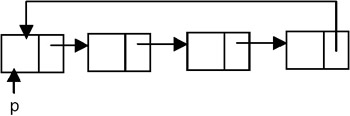Figure 20.16: List containing 2n nodes.

To split this list into two equal lists, it is required to traverse the list up to the nth node and store the link of the nth node, which is the address of (n+1)th node in the pointer, say q. After this, make the link field of the nth node point to the first node pointed to by p. Then traverse the list starting from the node pointed to by q up to the end. Then make the link field of the last node point to the node pointed to by q. The result of this is shown in Figure 20.17.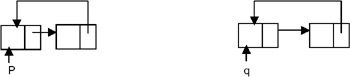Figure 20.17: Splitting of a circular list.

## MERGING OF TWO CIRCULAR LISTS

### Introduction

You can merge two lists into one list. The following program merges two circular lists.

Program

```
# include
# include
struct node
{
int data;
};

struct node *insert(struct node *p, int n)
{
struct node *temp;
/* if the existing list is empty then insert a new node as the
starting node */
if(p==NULL)
{
p=(struct node *)malloc(sizeof(struct node)); /* creates new node
data value passes
as parameter */

if(p==NULL)
{
printf("Error
");
exit(0);
}
p-> data = n;
p-> link = p; /* makes the pointer pointing to itself because it
is a circular list*/
}
else
{
temp = p;
/* traverses the existing list to get the pointer to the last node of
it */
temp-> link = (struct node *)malloc(sizeof(struct node)); /*
creates new node using
data value passes as
parameter and puts its
of last node of the
existing list*/
{
printf("Error
");
exit(0);
}
temp-> data = n;
}
return (p);
}
void printlist ( struct node *p )
{
struct node *temp;
temp = p;
printf("The data values in the list are
");
if(p!= NULL)
{
do
{
printf("%d	",temp->data);
} while (temp!= p);
}
else
printf("The list is empty
");
}
struct node *merge(struct node *p, struct node *q)
{
struct node *temp=NULL;
struct node *r=NULL;
r = p;
temp = p;
temp = q;
return(r);
}

void main()
{
int n;
int x;
struct node *start1=NULL ;
struct node *start2=NULL;
struct node *start3=NULL;

/* this will create the first circular list nodes*/
printf("Enter the number of nodes in the first list
");
scanf("%d",&n);
while ( n-- > 0 )
{
printf( "Enter the data value to be placed in a node
");
scanf("%d",&x);
start1 = insert ( start1, x );
}
printf("The first list is
");
printlist ( start1 );

/* this will create the second circular list nodes*/
printf("Enter the number of nodes in the second list
");
scanf("%d",&n);
while ( n-- > 0 )
{
printf( "Enter the data value to be placed in a node
");
scanf("%d",&x);
start2 = insert ( start2, x );
}
printf("The second list is:
");
printlist ( start2 );

start3 = merge(start1,start2);
printf("The resultant list is:
");
printlist(start3);
}
```

Explanation

In order to merge or concatenate the two non-empty circular lists pointed to by p and q, it is required to make the start of the resultant list p. Then the list pointed to by p is required to be traversed until its end, and the link field of the last node must become the pointer q. After that, the list pointed to by q is required to be traversed until its end, and the link field of the last node is required to be made p.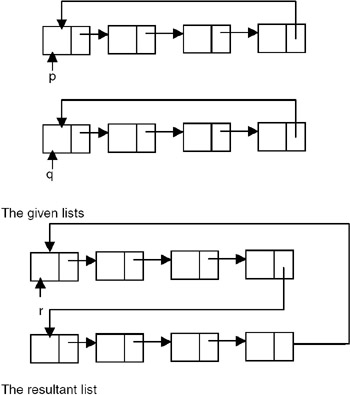## REVERSING THE DIRECTION OF LINKS IN A SINGLY LINKED CIRCULAR LIST

### Introduction

You can reverse the direction of links in the circular list. If you do so, each link should be reversed.

Program

```
# include
# include
struct node
{
int data;
};
/* A function to reverse a singly linked circular list */
struct node *reverselist(struct node *p)
{
struct node *temp;
struct node *prev = NULL;
struct node *curr;
if(p != NULL)
{
curr = p;
temp = curr;
{
prev = temp;
temp = curr;
}
p= temp;
}
return(p);
}

struct node *insert(struct node *p, int n)
{
struct node *temp;
/* if the existing list is empty then insert a new node as the
starting node */
if(p==NULL)
{
p=(struct node *)malloc(sizeof(struct node)); /* creates new node
data value passes
as parameter */

if(p==NULL)
{
printf("Error
");
exit(0);
}
p-> data = n;
p-> link = p; /* makes the pointer point to itself because it is
a circular list*/
}
else
{
temp = p;
/* traverses the existing list to get the pointer to the last node of
it */
temp-> link = (struct node *)malloc(sizeof(struct node)); /*
creates new node using
data value passes as
parameter and puts its
of last node of the
existing list*/
{
printf("Error
");
exit(0);
}
temp-> data = n;
}
return (p);
}
void printlist ( struct node *p )
{
struct node *temp;
temp = p;
printf("The data values in the list are
");
if(p!= NULL)
{
do
{
printf("%d	",temp->data);
} while (temp!= p);
}
else
printf("The list is empty
");
}
void main()
{
int n;
int x;
struct node *start = NULL ;
struct node *start1=NULL;
/* this will create at circular list */
printf("Enter the number of nodes in the list
");
scanf("%d",&n);
while ( n-- > 0 )
{
printf( "Enter the data value to be placed in a node
");
scanf("%d",&x);
start = insert ( start, x );
}
printf("The list is
");
printlist ( start );
start1 = reverselist(start);
printf("The reversed list is:
");
printlist(start1);
}
```

Explanation

To reverse the links of a singly linked circular list, the list is required to be traversed from the start node until a node is encountered whose link points to the start node (that is, the last node in the list). For this, it is required to maintain the pointers to the current node and the previous node. An additional temporary pointer pointing to the current node is also required to be maintained. Initially, the current, temporary, and previous pointers are set as follows:

1. Set the current as well as the temporary pointer to the start pointer.
2. Set the previous pointer to NULL.
3. The pointers are manipulated in each iteration as follows:

1. Advance the current pointer to make it point to the next node.
2. Set the link field of the node pointed to by the temporary pointer to the value of the previous pointer.
3. Make the previous pointer point to the node pointed to by the temporary pointer.
4. Make the temporary pointer point to the node pointed to by the current pointer.
5. When the last node is encountered, its link field is made to point to the previous node. After that, the link field of the node pointed to by the start pointer (first node) is made to point to this last node. And the start pointer is made to point to this last node. These manipulations are shown in the following diagrams.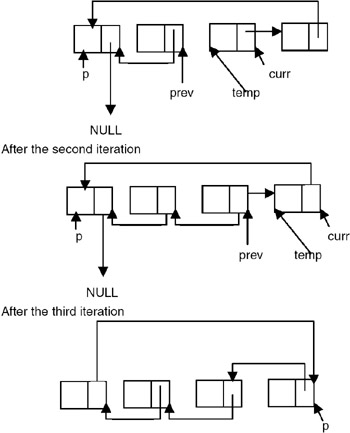### Introduction

The following are problems with singly linked lists:

1. A singly linked list allows traversal of the list in only one direction.
2. Deleting a node from a list requires keeping track of the previous node, that is, the node whose link points to the node to be deleted.
3. If the link in any node gets corrupted, the remaining nodes of the list become unusable.Figure 20.18: A doubly linked list maintained as chain.

If it is a circular list without a header node, the right link of the last node points to the first node. The left link of the first node points to the last node, as shown in Figure 20.19.Figure 20.19: A doubly linked list maintained as a circular list.

If it is a circular list with a header node, the left link of the first node and the right link of the last node point to the header node. The right link of the header node points to the first node and the left link of the header node points to the last node of the list, as shown in Figure 20.20.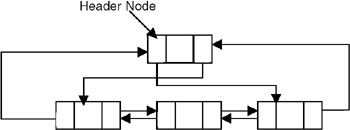Figure 20.20: A doubly linked list maintained as a circular list with a header node.

Therefore, the following representation is required to be used for the nodes of a doubly linked list.

```struct dnode
{
int data;
struct dnode *left,*right;
};
```

Program

A program for building and printing the elements of a doubly linked list follows:

``` # include
# include
struct dnode
{
int data;
struct dnode *left, *right;
};
struct dnode *insert(struct dnode *p, struct dnode **q, int n)
{
struct dnode *temp;
/* if the existing list is empty then insert a new node as the
starting node */
if(p==NULL)
{
p=(struct dnode *)malloc(sizeof(struct dnode)); /* creates new
node data value
passed as parameter */

if(p==NULL)
{
printf("Error
");
exit(0);
}
p->data = n;
p-> left = p->right =NULL;
*q =p;
}
else
{
temp = (struct dnode *)malloc(sizeof(struct dnode)); /* creates
new node using
data value passed as
parameter and puts its
*/
if(temp == NULL)
{
printf("Error
");
exit(0);
}
temp->data = n;
temp->left = (*q);
temp->right = NULL;
(*q)->right = temp;
(*q) = temp;
}
return (p);
}
void printfor( struct dnode *p )
{
printf("The data values in the list in the forward order are:
");
while (p!= NULL)
{
printf("%d	",p-> data);
p = p->right;
}
}
void printrev( struct dnode *p )
{
printf("The data values in the list in the reverse order are:
");
while (p!= NULL)
{
printf("%d	",p->data);
p = p->left;
}
}
void main()
{
int n;
int x;
struct dnode *start = NULL ;
struct dnode *end = NULL;
printf("Enter the nodes to be created
");
scanf("%d",&n);
while ( n-- > 0 )
{
printf( "Enter the data values to be placed in a node
");
scanf("%d",&x);
start = insert ( start, &end,x );
}
printf("The created list is
");
printfor ( start );
printrev(end);
}
```

Explanation

1. This program uses a strategy of inserting a node in an existing list to create it. For this, an insert function is used. The insert function takes a pointer to an existing list as the first parameter.
2. The pointer to the last node of a list is the second parameter. A data value with which the new node is to be created is the third parameter. This creates a new node using the data value, appends it to the end of the list, and returns a pointer to the first node of the list. Initially, the list is empty, so the pointer to the start node is NULL. When insert is called the first time, the new node created by the insert becomes the start node.
3. Subsequently, insert creates a new node that stores the pointer to the created node in a temporary pointer. Then the left link of the node pointed to by the temporary pointer becomes the last node of the existing list, and the right link points to NULL. After that, it updates the value of the end pointer to make it point to this newly appended node.
4. The main function reads the value of the number of nodes in the list, and calls insert that many times by going in a while loop, in order to get a doubly linked list with the specified number of nodes created.

## INSERTION OF A NODE IN A DOUBLY LINKED LIST

### Introduction

The following program inserts the data in a doubly linked list.

Program

``` # include
# include
struct dnode
{
int data;
struct node *left, *right;
};
struct dnode *insert(struct dnode *p, struct dnode **q, int n)
{
struct dnode *temp;
/* if the existing list is empty then insert a new node as the
starting node */
if(p==NULL)
{
p=(struct dnode *)malloc(sizeof(struct dnode)); /* creates new
node data value
passed as parameter */

if(p==NULL)
{
printf("Error
");
exit(0);
}
p-> data = n;
p-> left = p->right =NULL;
*q =p
}
else
{
temp = (struct dnode *)malloc(sizeof(struct dnode)); /* creates
new node using
data value passed as
parameter and puts its
*/
if(temp == NULL)
{
printf("Error
");
exit(0);
}
temp-> data = n;
temp->left = (*q);
temp->right = NULL;
(*q) = temp;
}
return (p);
}
void printfor( struct dnode *p )
{
printf("The data values in the list in the forward order are:
");
while (p!= NULL)
{
printf("%d	",p-> data);
p = p-> right;
}
}
/* A function to count the number of nodes in a doubly linked list */
int nodecount (struct dnode *p )
{
int count=0;
while (p != NULL)
{
count ++;
p = p->right;
}
return(count);
}

/* a function which inserts a newly created node after the specified
node in a doubly
struct node * newinsert ( struct dnode *p, int node_no, int value )
{
struct dnode *temp, * temp1;
int i;
if ( node_no <= 0 || node_no > nodecount (p))
{
printf("Error! the specified node does not exist
");
exit(0);
}
if ( node_no == 0)
{
temp = ( struct dnode * )malloc ( sizeof ( struct dnode ));
if ( temp == NULL )
{
printf( " Cannot allocate
");
exit (0);
}
temp -> data = value;
temp -> right = p;
temp->left = NULL
p = temp ;
}
else
{
temp = p ;
i = 1;
while ( i < node_no )
{
i = i+1;
temp = temp-> right ;
}
temp1 = ( struct dnode * )malloc ( sizeof(struct dnode));
if ( temp == NULL )
{
printf("Cannot allocate
");
exit(0);
}
temp1 -> data = value ;
temp1 -> right = temp -> right;
temp1 -> left = temp;
temp1->right->left = temp1;
temp1->left->right = temp1
}
return (p);
}
void main()
{
int n;
int x;
struct dnode *start = NULL ;
struct dnode *end = NULL;
printf("Enter the nodes to be created
");
scanf("%d",&n);
while ( n- > 0 )
{
printf( "Enter the data values to be placed in a node
");
scanf("%d",&x);
start = insert ( start, &end,x );
}
printf("The created list is
");
printfor ( start );
printf("enter the node number after which the new node is to be
inserted
");
scanf("%d",&n);
printf("enter the data value to be placed in the new node
");
scanf("%d",&x);
start=newinsert(start,n,x);
printfor(start);
}
```

Explanation

1. To insert a new node in a doubly linked chain, it is required to obtain a pointer to the node in the existing list after which a new node is to be inserted.
2. To obtain this pointer, the node number after which the new node is to be inserted is given as input. The nodes are assumed to be numbered as 1,2,3,…, etc., starting from the first node.
3. The list is then traversed starting from the start node to obtain the pointer to the specified node. Let this pointer be x. A new node is then created with the required data value, and the right link of this node is made to point to the node to the right of the node pointed to by x. And the left link of the newly created node is made to point to the node pointed to by x. The left link of the node which was to the right of the node pointed to by x is made to point to the newly created node. The right link of the node pointed to by x is made to point to the newly created node.

## DELETING A NODE FROM A DOUBLY LINKED LIST

### Introduction

The following program deletes a specific node from the linked list.

Program

``` # include
# include
struct dnode
{
int data;
struct dnode *left, *right;
};
struct dnode *insert(struct dnode *p, struct dnode **q, int n)
{
struct dnode *temp;
/* if the existing list is empty then insert a new node as the
starting node */
if(p==NULL)
{
p=(struct dnode *)malloc(sizeof(struct dnode)); /* creates new node
data value
passed as parameter */

if(p==NULL)
{
printf("Error
");
exit(0);
}
p-> data = n;
p-> left = p->right =NULL;
*q =p;
}
else
{
temp = (struct dnode *)malloc(sizeof(struct dnode)); /* creates
new node using
data value passed as
parameter and puts its
*/
if(temp == NULL)
{
printf("Error
");
exit(0);
}
temp-> data = n;
temp->left = (*q);
temp->right = NULL;
(*q)->right = temp;
(*q) = temp;
}
return (p);
}
void printfor( struct dnode *p )
{
printf("The data values in the list in the forward order are:
");
while (p!= NULL)
{
printf("%d	",p-> data);
p = p-> right;
}
}
/* A function to count the number of nodes in a doubly linked list */
int nodecount (struct dnode *p )
{
int count=0;
while (p != NULL)
{
count ++;
p = p->right;
}
return(count);
}

/* a function which inserts a newly created node after the specified
node in a doubly
struct dnode * delete( struct dnode *p, int node_no, int *val)
{
struct dnode *temp ,*prev=NULL;
int i;
if ( node_no <= 0 || node_no > nodecount (p))
{
printf("Error! the specified node does not exist
");
exit(0);
}
if ( node_no == 0)
{
temp = p;
p = temp->right;
p->left = NULL;
*val = temp->data;
return(p);
}
else
{
temp = p ;
i = 1;
while ( i < node_no )
{
i = i+1;
prev = temp;
temp = temp-> right ;
}
prev->right = temp->right;
if(temp->right != NULL)
temp->right->left = prev;
*val = temp->data;
free(temp);
}
return (p);
}

void main()
{
int n;
int x;
struct dnode *start = NULL ;
struct dnode *end = NULL;
printf("Enter the nodes to be created
");
scanf("%d",&n);
while ( n-- > 0 )
{
printf( "Enter the data values to be placed in a node
");
scanf("%d",&x);
start = insert ( start, &end,x );
}
printf("The created list is
");
printfor ( start );
printf("enter the number of the node which is to be deleted
");
scanf("%d",&n);
start=delete(start,n,&x);
printf("The data value of the node deleted from list is :
%d
",x);
printf("The list after deletion of the specified node is :
");
printfor(start);
}
```

Explanation

1. To delete a node from a doubly linked chain, it is required to obtain a pointer to the node in the existing list that appears to the left of the node which is to be deleted.
2. To obtain this pointer, the node number which is to be deleted is given as input. The nodes are assumed to be numbered 1,2,3,…, etc., starting from the first node.
3. The list is then traversed starting from the start node to obtain the pointer to the specified node. Let this pointer be x. A pointer to the node to the right of the node x is also obtained. Let this be pointer y (this is a pointer to the node to be deleted). The right link of the node pointed to by x is the node pointing to the node to which the right link of the node pointed to by y points. The left link of the node to the right of the node pointed to by y is made to point to x. The node pointed to by y is then freed.

## APPLICATION OF DOUBLY LINKED LISTS TO MEMORY MANAGEMENT

### Introduction

A doubly linked list is used to maintain both the list of allocated blocks and the list of free blocks by the memory manager of the operating system. To keep track of the allocated and free portions of memory, the memory manager is required to maintain a linked list of allocated and free segments. Each node of this list contains a starting address, size, and status of the segment. This list is kept sorted by the starting address field to facilitate the updating, because when a process terminates, the memory segment allocated to it becomes free, and so if any of the segments are freed, then they can be merged with the adjacent segment, if the adjacent segment is already free. This requires traversal of the list both ways to find out whether any of the adjacent segments are free. So this list is required to be maintained as a doubly linked list. For example, at a particular point in time, the list may be as shown in Figure 20.21.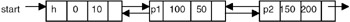Figure 20.21: Before termination of process p1.

If the process p1 terminates, it is required to be modified as shown in Figure 20.22.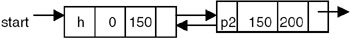Figure 20.22: After termination of process p1.

1. A linked list is a dynamic data structure that can grow and shrink based on need.
2. The elements are not necessarily at a fixed distance apart.
3. In a linked list, the elements are placed in non-contiguous blocks of memory, and each block is linked to its previous block.
4. To link the next element to the previous element, the address of the next element is stored in the previous element itself.
5. Insertion or deletion at any arbitrary position in the linked list can be done easily, since it requires adjustment of only a few pointers.
6. Linked lists can be used for manipulation of symbolic polynomials.
7. A linked list is suitable for representation of sparse matrices.
8. A circular list is a list in which the link field of the last node is made to point to the start/first node of the list
10. The left link of the node in a DLL is made to point to the previous node, whereas the right link is made to point to the next node.
11. A DLL can be traversed in both directions.
12. Having two pointers in a DLL provides safety, because even if one of the pointers get corrupted, the node still remains linked.
13. Deleting a particular node from a list, therefore, does not require keeping track of the previous node in a DLL.

Exercises

1. Write a C program to delete a node with the minimum value from a singly linked list.
2. Write a C program that will remove a specified node from a given doubly linked list and insert it at the end of the list.
3. Write a C program to transform a circular list into a chain.
4. Write a C program to merge two given lists A and B to form C in the following manner:

The first element of C is the first element of A and the second element of C is the first element of B. The second elements of A and B become the third and fourth elements of C, and so on. If either A or B gets exhausted, the remaining elements of the other are to be copied to C.

5. Write a C program to delete all occurrences of x from a given singly linked list.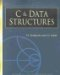C & Data Structures (Charles River Media Computer Engineering)
ISBN: 1584503386
EAN: 2147483647
Year: 2006
Pages: 232